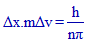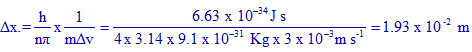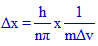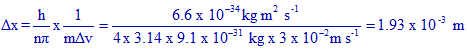# UNCERTAINTY IN POSITION - VELOCITY - MOMENTUM IIT JEE - NEET

## Question: Uncertainty in the position of an electron (mass = 9.1 x 10-34 kg) moving with a velocity 300 m s-1 , accurate up to 0.001%, will be: (h= 6.63 x 10-34 J s)

#### (AIEEE 2006)

a) 19.2 x 10-2

b) 5.76 x 10-2

c) 1.92 x 10-2

d) 3.84 x 10-2 m

According to Heisenberg's uncertainty principle, the product of uncertainties in position (Δx) and velocity (Δv) is always equal to or greater than h/nπ.

i.e.What is the meaning of "accurate up to 0.001%"?

It indicates that the uncertainty in velocity is 0.001% of actual value, 300 m s-1.

i.e.

uncertainty in velocity (Δv) = 300 x 0.001 / 100 = 3 x 10-3  m s-1

### Solution:

The uncertainty in position (Δx) can be calculated as follows:Note: n = 4 for electron.

Conclusion:

The correct option is "c".

## Related questions

### 1) If the uncertainties in velocities of two particles A and B with mass 1.0 x 10-27 kg and 1.0 x 10-31 kg respectively are same, the ratio of uncertainties in the positions of A and B is:

(EAMCET 2011-E)

a) 1000 :1

b) 10,000:1

c) 1: 1000

d) 1:10,000

Logic & solution:

The uncertainty in position, Δx is given by the expression:The ratio of uncertainties in positions for A and B is:Note: h/nπ  is constant and Δv values are same.

Conclusion:

Correct option is "c".

### 2) The uncertainties in the velocities of two particles A and B are 0.05 and 0.02 m s-1 respectively. The mass of B is five times to that of mass A. What is the ratio of uncertainties?

(EAMCET 2006-E)

a) 2

b) 0.25

c) 4

d) 1

Logic & solution:

The uncertainty in position, Δx is given by the expression:The ratio of uncertainties in positions for A and B is:Note: mB = 5mA

Conclusion:

Correct option is "a".

### 3) Uncertainty in position of a particle of 25 g in space is 10-5 m. Hence, uncertainty in velocity (m s-1) is: ( Planck’s constant, h = 6.6 x 10-34 J s)

(AIEEE 2002)

a) 2.1 x 10-28

b) 2.1 x 10-34

c) 0.5 x 10-34

d) 5.0 x 10-24

Logic & solution:

The uncertainty in velocity, Δv is given by the expression:Conclusion:

Correct option is "a".

### 4) In an atom, an electron is moving with a speed of 600 m/s with an accuracy of 0.005%. Certainty with which the position of the electron can be located is: (h=6.6 x 10-34 kg m2 s-1; mass of electron, em = 9.1 x 10-31 kg )

(AIEEE 2009)

1) 1.52 x 10-4

2) 5.10 x 10-3

3) 1.92 x 10-3

4) 3.84 x 10-3 m

Logic & solution:

Since the accuracy in velocity is 0.005%, uncertainty in velocity, Δv = 600 x 0.005 / 100 = 3 x 10-2 m s-1.

Uncertainty in position will be:Conclusion:

Correct option is "3".

## Homework

1) State Heisenberg's uncertainty principle.

2) What will be the uncertainty in momentum of electron if the uncertainty in its position is zero?

Jump to De broglie Wavelength - IIT JEE - NEET - IT JAM solved problems